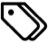## Creating Bandpass Bessel Filter with MATLABMatlab | programming | research | bitbucket

Bessel filters are incredibly useful in numerical analysis, especially for acoustic-type waveforms. This is because analog Bessel filters are characterized by almost constant group delay over any frequency band, and this means that the shape of waves does not change when passed through such a filter.

Well, MATLAB provides some of the building blocks required to create a bandpass analog filter, but does not actually combine the pieces to make a usable filter function.

I created a function for my own research (sourced from pieces I found elsewhere, but it’s been too long—I don’t remember where I found each piece, sorry!), and can be found at my MATLAB repository, specifically, here.

Here’s the documentation that I included with the function:

``````besselfilter. Function to implement a bandpass Bessel Filter.

[filtData, b, a] = besselfilter(order,low,high,sampling,data)

Inputs:

- order:      Number of poles in the filter. Scalar numeric value.
Eg.: 4
- low:        Lower frequency bound (Hz). Scalar numeric value.
Eg.: 50000 (= 50kHz)
- high:       Upper frequency bound (Hz). Scalar numeric value.
Eg.: 1000000 (= 1MHz)
- sampling:   Sampling frequency (Hz). Scalar numeric value.
Eg.: 25000000 (= 25MHz)
- data:       Input data. Numeric vector.
Eg.: data vector of size (n x 1)

Output:

- filtData:   Output filtered data. Numeric vector.
Eg.: data vector of size (n x 1)
- b, a:       Transfer function values for the filter. Scalar numeric.
``````

## MATLAB repositoryMatlab | programming | research | bitbucket | github

I’ve been using MATLAB for quite a few years now, using it both for my own research as well for work at VTTI. Well, I decided to share some of the code that I’ve been writing, which may come in handy to others in the same field. I’ve long appreciated the help I’ve received from the larger MATLAB community, and I thought I should start contributing as well. :)